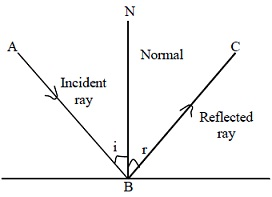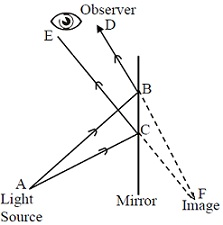#### Reflection at Plane Surfaces, Physics tutorial

Introduction:

When the wave strikes the smooth plane (flat) surface it may be reflected / refracted (bent) or absorbed or any combination of these. The significant application of total reflection off a plane surface is summarized in the law of reflection.

Laws of reflection:In figure given above, i is an angle of incident and r is an angle of reflection. It shows the ray of light AB which is incident on surface of the plane mirror at the angle of incident i from normal to mirror. BC is the ray of light reflected from surface of the mirror, thus is called as reflected ray. Angle formed by reflected ray with normal is r known as angle of reflection. The incident ray, reflected ray and normal to mirror at the point of incidence all lie in same plane. This is the first law of reflection.

It has been experimentally found that angle i = angle r .......................Eq.1

Equation 1 signifies that angle of incident is always equal to the angle of reflection. This has given rise to what is called as second law of reflection.

As a result the laws of reflection can be summarized below:

1st Law

The incident ray, the reflected ray and the normal at the point of incidence all lie in the same plane.

2nd Law

Angle of incident equals angle of reflection.

Reflection at Plane Surfaces:

When light is reflected from the surface i.e. smooth or polished it may serve as a mirror and generate a reflected image. If mirror is flat, or plane, image of object seems to lie behind mirror at the distance equal to distance between the object and the surface of the mirror. In figure given below, light source is object A, and point on A sends out rays in all directions. Two rays which strike mirror at B and C, are reflected as rays BD and CE. To the observer in front of mirror, these rays appear to come from point F behind.Formation of Image by Plane Mirror:

In the mirror, it follows from laws of reflection that CF and BF form same angle with surface of mirror, as do AC and AB. If surface of reflection is rough, then normal to different points of surface lie in random directions in that situation, rays which may lie in same plane when they emerge from the point source however lie in random planes of incidence and thus of reflection, and are scattered and can't form image.

Image Formed by Plane Mirror:

The real image is the one formed by actual intersection of light rays, and can be captured on the screen. The virtual image is that created by imaginary intersection of light rays and cann't be formed or captured on screen.

Main features of images formed by plane mirror are given below:

i) It is upright, i.e., image is oriented in same direction as the object.

ii) It is virtual, i.e., it can't be received on screen.

iii) It is of the same size as object.

iv) It is laterally inverted.

Tutorsglobe: A way to secure high grade in your curriculum (Online Tutoring)

Expand your confidence, grow study skills and improve your grades.

Since 2009, Tutorsglobe has proactively helped millions of students to get better grades in school, college or university and score well in competitive tests with live, one-on-one online tutoring.

Using an advanced developed tutoring system providing little or no wait time, the students are connected on-demand with a tutor at www.tutorsglobe.com. Students work one-on-one, in real-time with a tutor, communicating and studying using a virtual whiteboard technology.  Scientific and mathematical notation, symbols, geometric figures, graphing and freehand drawing can be rendered quickly and easily in the advanced whiteboard.

Free to know our price and packages for online physics tutoring. Chat with us or submit request at info@tutorsglobe.com